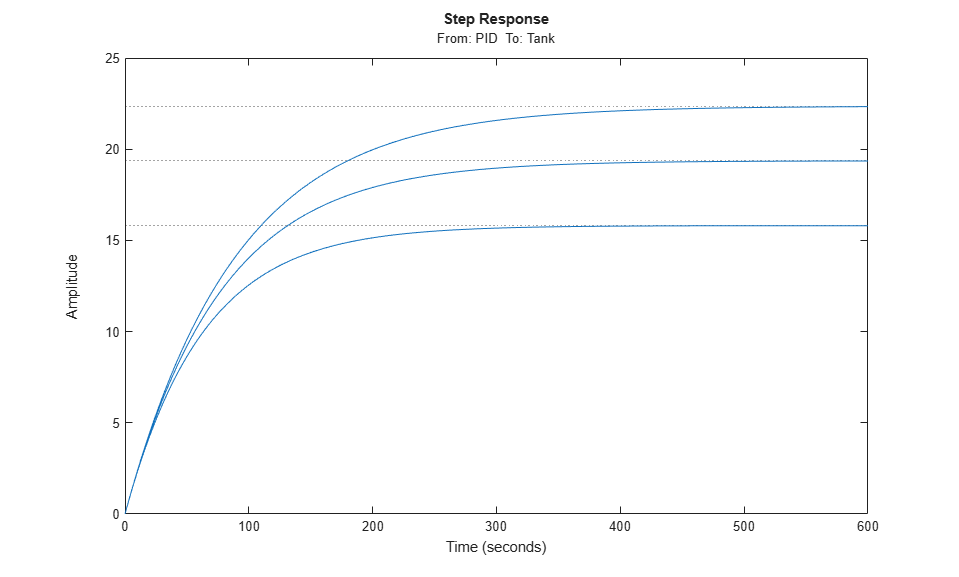# Vary Operating Points and Obtain Multiple Transfer Functions Using slLinearizer Interface

This example shows how to use the `slLinearizer` interface to batch linearize a Simulink® model. You linearize a model at multiple operating points and obtain multiple open-loop and closed-loop transfer functions from the model.

You can perform the same analysis using the `linearize` command. However, when you want to obtain multiple open-loop and closed-loop transfer functions, especially for models that are expensive to compile repeatedly, `slLinearizer` can be more efficient.

### Create slLinearizer Interface for Model

Open the model.

```mdl = 'watertank'; open_system(mdl); ```Use the `slLinearizer` command to create the interface.

```sllin = slLinearizer(mdl) ```
``` slLinearizer linearization interface for "watertank": No analysis points. Use the addPoint command to add new points. No permanent openings. Use the addOpening command to add new permanent openings. Properties with dot notation get/set access: Parameters : [] OperatingPoints : [] (model initial condition will be used.) BlockSubstitutions : [] Options : [1x1 linearize.LinearizeOptions] ```

The command-window display shows information about the `slLinearizer` interface. In this interface, the `OperatingPoints` property display shows that no operating point is specified.

### Specify Multiple Operating Points for Linearization

You can linearize the model using trimmed operating points, the model initial condition, or simulation snapshot times. For this example, use trim points that you obtain for varying water-level reference heights.

```opspec = operspec(mdl); opspec.States(2).Known = 1; opts = findopOptions('DisplayReport','off'); h = [10 15 20]; for ct = 1:numel(h) opspec.States(2).x = h(ct); Href = h(ct); ops(ct) = findop(mdl,opspec,opts); end sllin.OperatingPoints = ops; ```

Here, `h` specifies the different water-levels. `ops` is a 1 x 3 array of operating point objects. Each entry of `ops` is the model operating point at the corresponding water level. Configure the `OperatingPoints` property of `sllin` with `ops`. Now, when you obtain transfer functions from `sllin` using the `getIOTransfer`, `getLoopTransfer`, `getSensitivity`, and `getCompSensitivity` functions, the software returns a linearization for each specified operating point.

Each trim point is only valid for the corresponding reference height, represented by the `Href` parameter of the Desired Water Level block. So, configure `sllin` to vary this parameter accordingly.

```param.Name = 'Href'; param.Value = h; sllin.Parameters = param; ```

### Analyze Plant Transfer Function

In the `watertank` model, the Water-Tank System block represents the plant. To obtain the plant transfer function, add the input and output signals of the Water-Tank System block as analysis points of `sllin`.

```addPoint(sllin,{'watertank/PID Controller','watertank/Water-Tank System'}) sllin ```
``` slLinearizer linearization interface for "watertank": 2 Analysis points: -------------------------- Point 1: - Block: watertank/PID Controller - Port: 1 Point 2: - Block: watertank/Water-Tank System - Port: 1 No permanent openings. Use the addOpening command to add new permanent openings. Properties with dot notation get/set access: Parameters : [1x1 struct], 1 parameters with sampling grid of size 1x3 "Href", varying between 10 and 20. OperatingPoints : [1x3 opcond.OperatingPoint] BlockSubstitutions : [] Options : [1x1 linearize.LinearizeOptions] ```

The first analysis point, which originates at the outport of the PID Controller block, is the input to the Water-Tank System block. The second analysis point is the output of the Water-Tank System block.

Obtain the plant transfer function from the input of the Water-Tank System block to the block output. To eliminate the effects of the feedback loop, specify the block output as a temporary loop opening.

```G = getIOTransfer(sllin,'PID','Tank','Tank'); ```

In the call to `getIOTransfer`, `'PID'`, a portion of the block name `'watertank/PID Controller'`, specifies the first analysis point as the transfer function input. Similarly, `'Tank'`, a portion of the block name `'watertank/Water-Tank System'`, refers to the second analysis point. This analysis point is specified as the transfer function output (third input argument) and a temporary loop opening (fourth input argument).

The output, `G`, is a 1 x 3 array of continuous-time state-space models.

Plot the step response for `G`.

```stepplot(G); ```The step response of the plant models varies significantly at the different operating points.

### Analyze Closed-Loop Transfer Function

The closed-loop transfer function is equal to the transfer function from the reference input, originating at the Desired Water Level block, to the plant output.

Add the reference input signal as an analysis point of `sllin`.

```addPoint(sllin,'watertank/Desired Water Level'); ```

Obtain the closed-loop transfer function.

```T = getIOTransfer(sllin,'Desired','Tank'); ```

The output, `T`, is a 1 x 3 array of continuous-time state-space models.

Plot the step response for `T`.

```stepplot(T); ```Although the step response of the plant transfer function varies significantly at the three trimmed operating points, the controller brings the closed-loop responses much closer together at all three operating points.

### Analyze Sensitivity at Plant Output

```S = getSensitivity(sllin,'Tank'); ```

The software injects a disturbance signal and measures the output at the plant output. `S` is a 1 x 3 array of continuous-time state-space models.

Plot the step response for `S`.

```stepplot(S); ```The plot indicates that both models can reject a step disturbance at the plant output within 40 seconds.